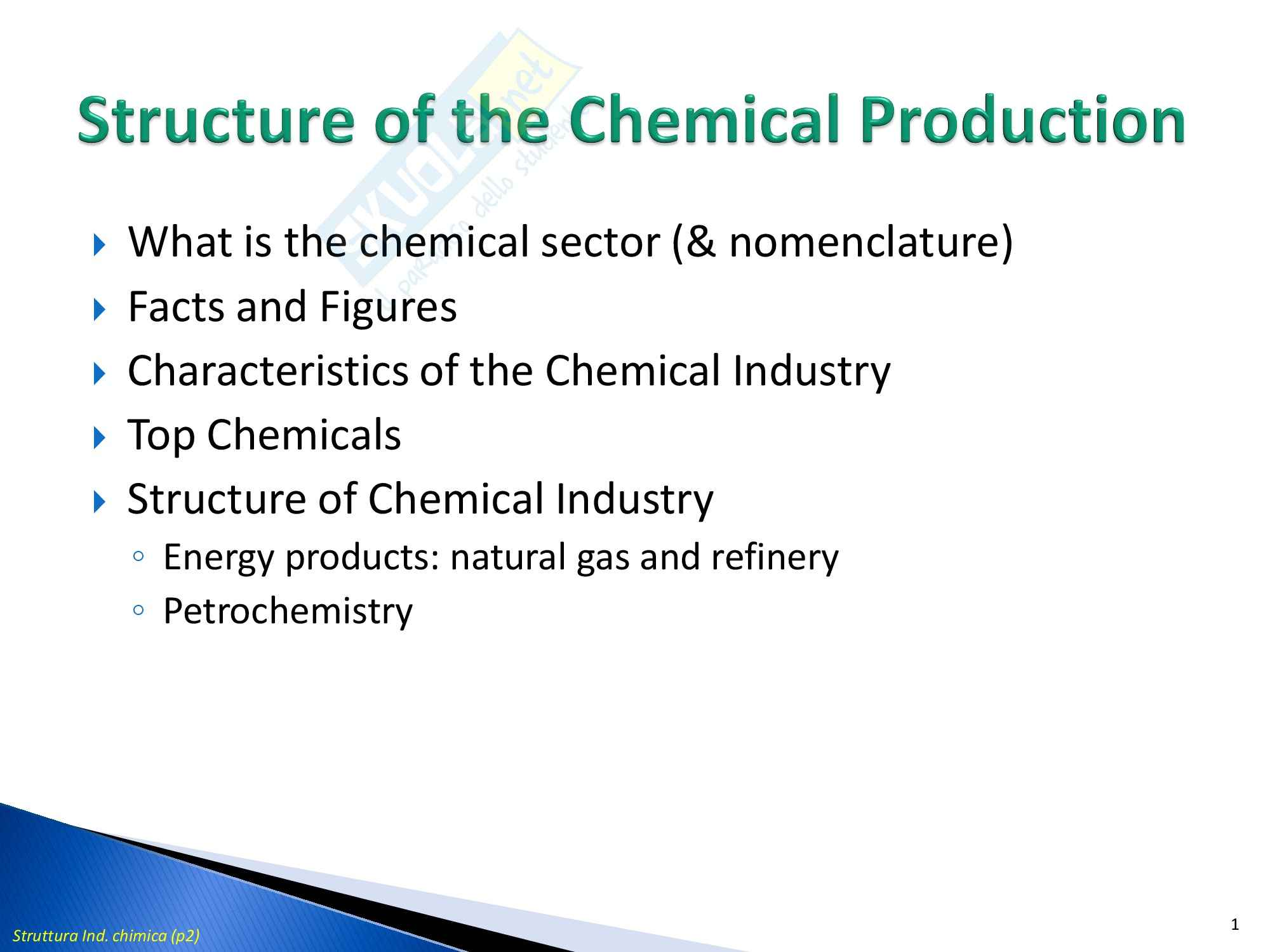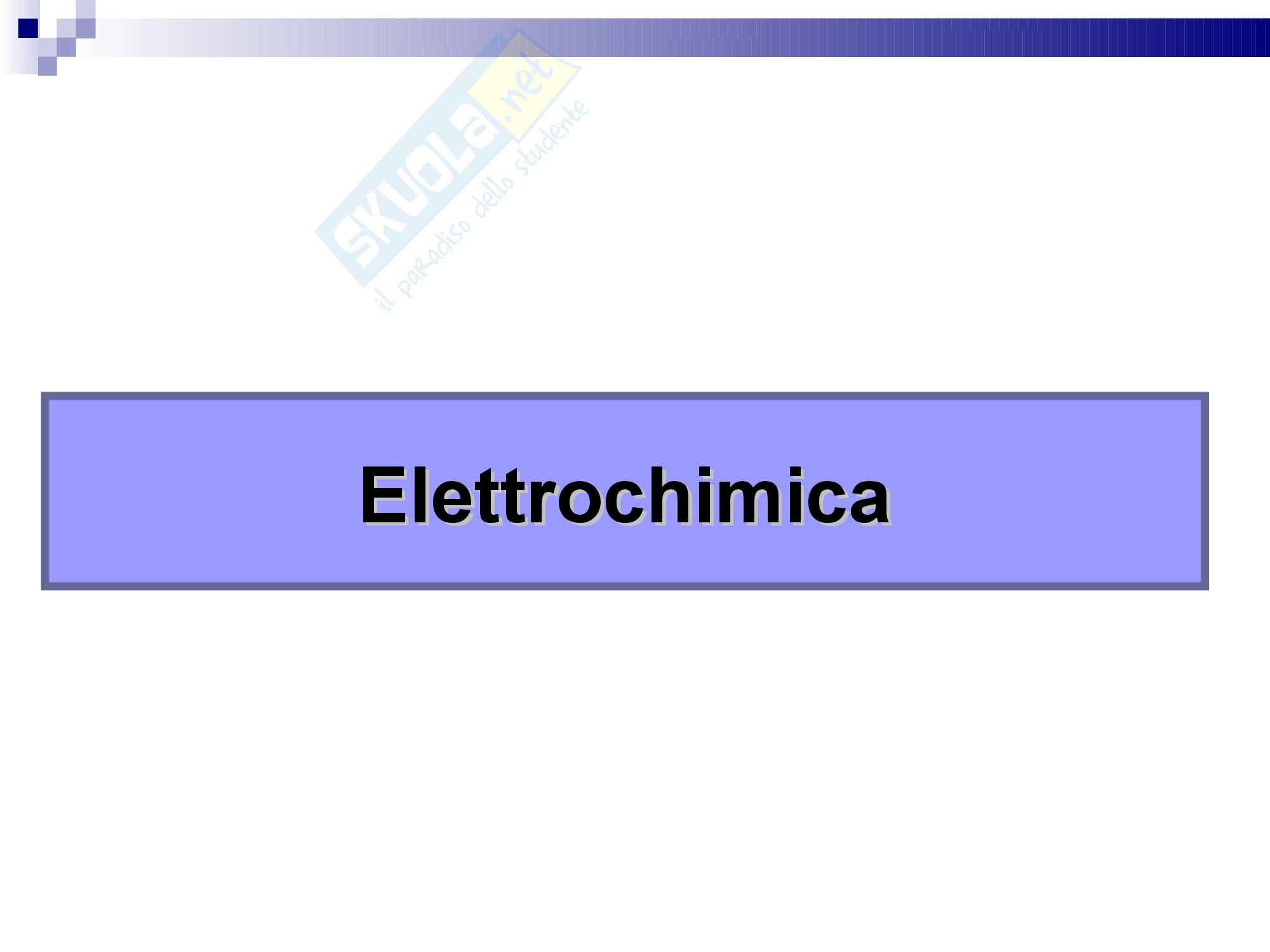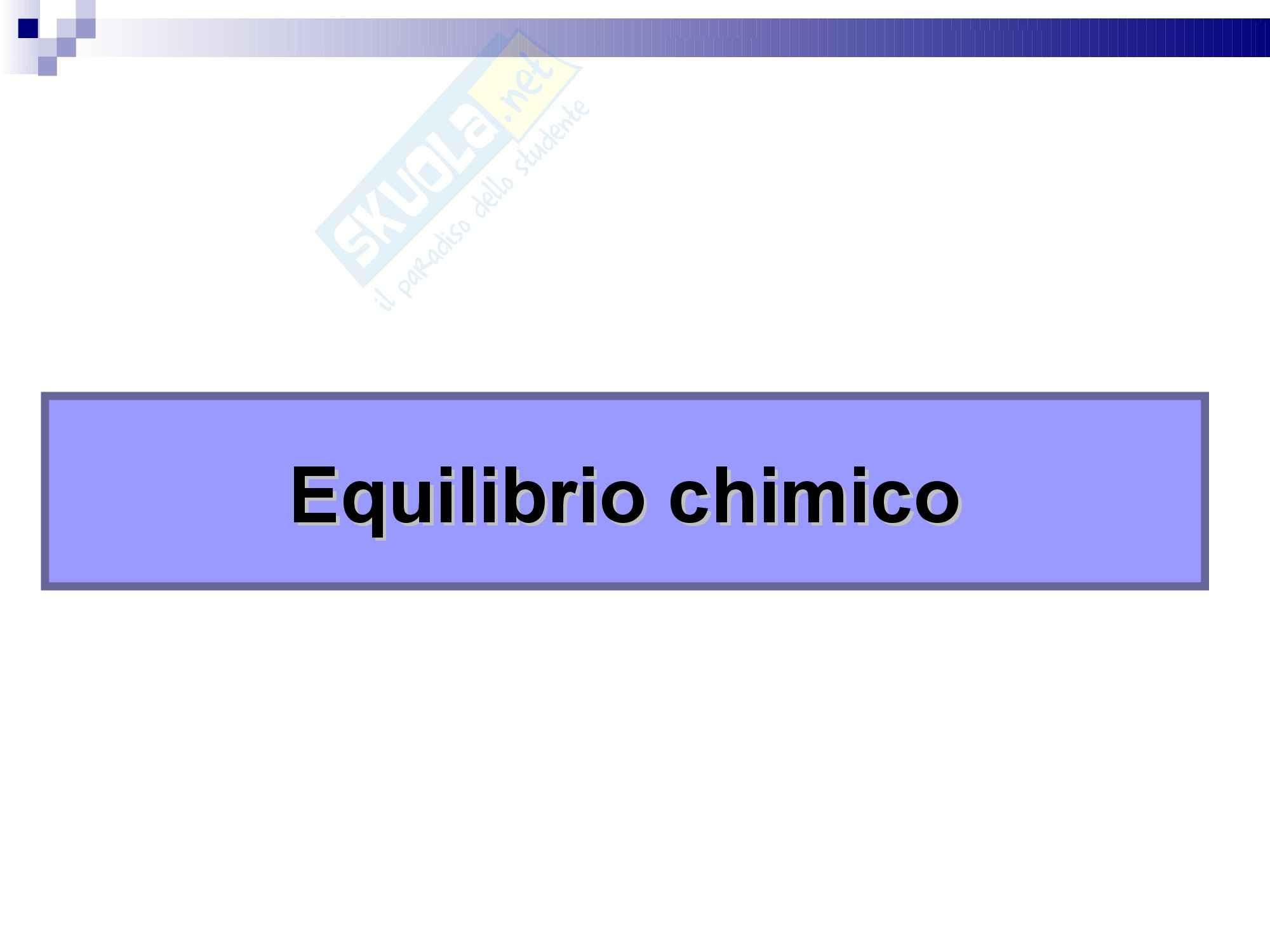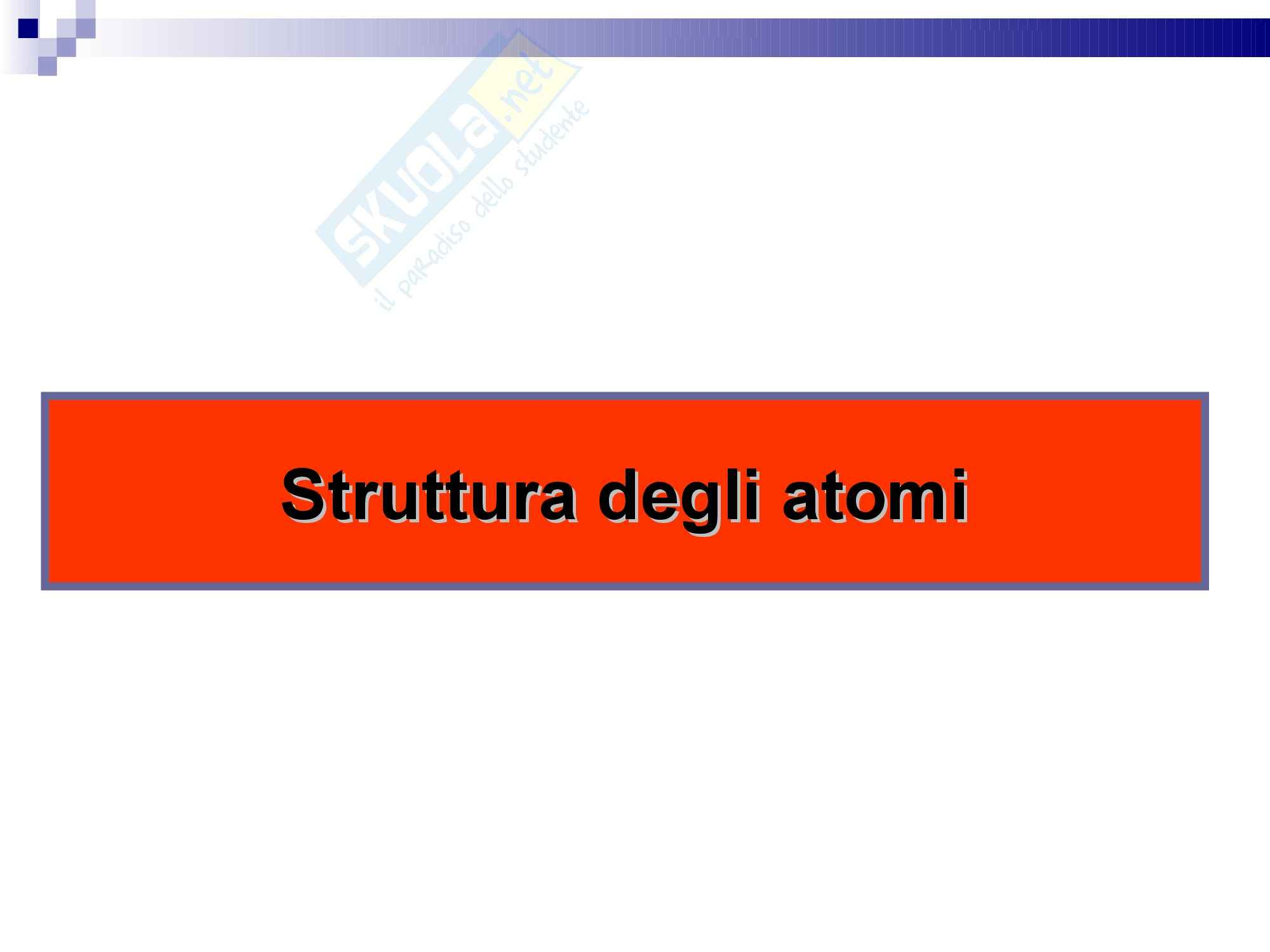Che materia stai cercando?

# Chimica industriale - struttura dell'industria chimica

Appunti di Chimica industriale per il corso della professoressa Perathoner. Gli argomenti trattati sono i seguenti: introduction to the chemical production, tools for industrial chemistry, the concept of flowsheet, the flowsheet is the “road-map’’ of a process.

Esame di Chimica industriale docente Prof. S. Perathoner

Anteprima

### ESTRATTO DOCUMENTO

gas-liq, exothermic reaction gas-gas, high exothermic

UniME 27

Mass and heat balances

Base concepts

UniME 28

Quantification of mass/heat transfer 1/..

Chemical processes may be classified as batch, continuous or semi-batch

▸ and as either steady-state or transient. Although the procedure required

for performing mass, or material, balances depends on the type of

process, most of the concepts translate directly to all types.

The general rule for mass balance in a system box (a box drawn around

▸ the complete process or the part of the process of interest) is:

input + generation - output - consumption = accumulation

where,

▸ input is the material entering through the system box. This will include feed and makeup

◦ streams;

generation is the material produced within the system, such as the reaction products in a

◦ reactor;

output is the material that leaves through the system boundaries. These will typically be

◦ the product streams of the process;

consumption is the material consumed within the system, such as the reactants in a

◦ reactor;

accumulation is the amount of material that builds up within the system.

UniME ◦ 29

Quantification of mass/heat transfer 2/..

In a steady-state continuous process, the accumulation should always be

▸ zero, which leads to a more simple mass balance equation:

input + generation = output + consumption

In the case of systems with no reaction, where mass is neither generated

▸ nor consumed, the result is even simpler:

input = output

Example 1: Mass Balance on a Continuous Distillation Process

▸ Suppose that a 1000 kmol h-1 feed stream, consisting of 30.0% by mole n-

◦ pentane and the remainder n-hexane, is to be separated into 95.0% pure

pentane and 95.0% pure hexane streams using a distillation column.

Determine the flow rates of the output streams through the use of mass

UniME 30

pentane has a lower boiling point than hexane.

first step is to draw and label a flowsheet

• diagram indicating the process steps and all

the streams

F is the feed stream, D the distillate or tops

• product stream (which will be primarily

pentane, the lighter of the two species), B

the bottoms product stream (primarily

hexane), p refers to pentane and h to

mole fractions hexane

input = output

• we have two unknowns. Therefore, we

• need to generate two independent

total and equations that will allow us to solve for

partial mass these unknowns (degrees of freedom)

balances

UniME 31

Mass Balance on a Process with Reaction

Suppose an initially empty tank is filled with 1000 mol of ethane and the

▸ remainder with air. A spark is used to ignite this mixture and the following

combustion reaction takes place:

2C2H6 + 7O2 4CO2 + 6H2O

Assume that the amount of air provides twice the stoichiometric

▸ requirement of oxygen for this reaction, and that air is composed of 79%

nitrogen and the remainder oxygen. Suppose that the reaction reaches a

90% conversion. What is the composition of the mixture in the tank at the

end of the reaction?

E for ethanol, O for oxygen,

N for nitrogen, C for carbon

dioxide and W for water

UniME input + generation = output + consumption 32

g is used to indicate the amount generated and the subscript c indicates the amount consumed

As there are five species involved, five mass balance equations can be defined.

• We have 19 unknown variables. As we have just defined 5 equations, we have

• 14 degrees of freedom remaining. To solve this problem, therefore, we need to

define at least 14 more equations.

We can write down new equations relating the unknown and known variables by

• making use of the stoichiometric coefficients This set of nine equations

reduces the degrees of

freedom to 5

UniME 33

Further equations can be defined on the basis of the specifications

of the feed and the conversion of the reaction:

The result is that we have

19 equations and 19

unknowns giving zero

degrees of freedom.

Given nl,E = 1000 mol, the initial amount of ethane

UniME 34

1/..

Energy balances

Energy balances can be treated in much the same way as

▸ material balances

Energy may be transferred between a system and its

▸ surroundings in two ways:

As heat, or energy that flows as a result of a temperature difference between a

◦ system and its surroundings. The direction of flow is always from the higher

temperature to the lower. Heat is defined as positive when it is transferred to

the system from its surroundings.

As work, or energy that flows in response to any driving force other than a

◦ temperature difference. For example, if a gas in a cylinder expands and moves a

piston against a restraining force, the gas does work on the piston. Energy is

transferred as work from the gas to its surroundings, including the piston.

Positive work means work done by the system on its surroundings, although

this convention is sometimes not followed and one should be careful to note

the convention used by other people.

UniME 35

the full energy balance equation is

▸ 2/..

As internal energy, U, is typically difficult to measure or estimate, enthalpy is instead used.

Specific enthalpy (enthalpy per unit mass), denoted by H, is defined as

UniME 36

In working with changes of energy, it is often useful to choose a reference 3/..

state, a state in which one of the quantities is assumed to be zero.

UniME (A) 37

Energy Balance on a Distillation Column 1/..

We consider the distillation unit introduced before, updated

▸ with temperature information for each of the streams. The

temperatures have been estimated using a physical property

estimation system. More streams are included. Specifically,

the vapour stream, V, from the top of the column to the

condenser and the liquid reflux stream, L, from the condenser

back into the column.

The relationship between the liquid reflux stream back into

▸ the column and the actual distillate product stream (D) is

given by the reflux ratio (typical value between 1.3 and 1.6;

1.6 in this case):

UniME Neglecting the effect of pressure on enthalpy, estimate the

▸ rate at which heat must be supplied 38

2/..

To determine this amount, we will

need to determine the change in

enthalpy of the output streams

relative to the feed stream and the

amount of cooling done in the

condenser

specific value

to determine

UniME 39

3/..

UniME (B) 40

4/..

The difference between the

enthalpies is primarily due to the calculated using eq. (A)

heats of vapourisation, but there and data in Table (B)

is a small contribution from the

drop in temperature across the

condenser

UniME (C) 41

Using the specific enthalpies in Table (C) to get the actual stream enthalpies, allows us 5/..

to then solve for the remaining three unknowns, with the following results

Looking at the system box 1, there are three streams, the feed stream and the two

output streams, and one heat input,

the energy balance around the column:

with unknowns Qr and the enthalpies of the feed and bottoms streams

the latter can be calculated as before, to finally obtain

UniME 42

Kinetics and thermodynamics

Base concepts

UniME 43

Kinetics and thermodynamics of reactions

DEFINITIONS 1/..

extend of reaction

▸ the change in the number of moles of any reaction compound

◦ (reactant or product) due to the chemical reaction divided by

its stoichiometric coefficient.

Conversion.

UniME ▸ The conversion of a reactant A (XA) is the fraction of reactant

◦ 44

limiting reagent

Kinetics and thermodynamics of reactions

DEFINITIONS 2/..

Yield (YK) molar (or by weight)

▸ amount of K produced expressed as a fraction of the

◦ maximum amount of K (according to the stoichiometry) that

could be produced. This is the amount of reactant A converted

to the specific product:

Selectivity:

▸ fraction of the converted reactant A (not the initial A) that is

◦ converted to the specific product.

Productivity

▸ moles (or weight) of product produced per time (h) and per

UniME volume (or mass catalyst) of reactor

dimensionally equivalent to rate, 45

BUT it is NOT a reaction rate

Reaction rate

The reaction rate (r) is the change of the extent of the

▸ reaction per unit time per unit volume.

for constant density this is

equal to dC/dt (C =

concentration).

In a reaction network of R reactions, the total change

▸ in the number of moles of a reaction component i is

due to many reactions and given by

UniME 46

Classification of reactions

UniME 47

Chemical Reaction Thermodynamics 1/..

for reversible reactions, at equilibrium, the rate of

▸ reaction from left to right is equal to the rate of the

reaction from right to left, so that the net overall

reaction rate is zero, i.e. nothing changes.

A + B C + D

The equilibrium conversion is the conversion of a

▸ reversible reaction at its equilibrium. This is the

maximum conversion that can be achieved.

the equilibrium can be shifted by subtracting one component,

◦ for example with a membrane

equilibrium constant (gas phase)

UniME R is the universal

gas constant. 48

Chemical Reaction Thermodynamics 2/..

Temperature Dependence of KP and Equilibrium

▸ Conversion.

UniME For ideal gases, KP is

independent of pressure 49

Effect of conc. on reaction rate

UniME 50

Rate expression – Gas phase reaction

UniME 51

Effect of temperature

Arrhenius plot

UniME 52

Heat of reaction

UniME 53

Thermodynamics

Equilibrium constant Reaction entropy

= ∆ = ∆ − ∆

o o o

RT ln K G (

T ) H (

T ) T S

eq Reaction enthalpy

∑ ν

= ∆ o

G (

T )

i f ,

i

i

(J/mol K)

0 0

∆ ∆

= −

## A A

ln K A R RT Adsorption enthalpy,<0

(J/mol)

UniME atm-1 54

Reaction rates

(aq) (l) (aq) (aq)

C4H9Cl + H2O C4H9OH + HCl



A plot of concentration vs.

▸ time for this reaction

yields a curve like this.

The slope of a line

▸ tangent to the curve at

any point is the

instantaneous rate at that

time.

UniME 55

Rate laws

A rate law shows the relationship between the

▸ reaction rate and the concentrations of reactants.

For gas-phase reactants use PA instead of [A].

Exponents tell the order of the reaction with respect to

▸ each reactant.

The overall reaction order can be found by adding the

◦ exponents on the reactants in the rate law.

UniME 56

Integrated Rate Laws 1/..

Consider a simple 1st order rxn: A B

Differential form:

How much A is left after time t? Integrate:

UniME 57

Integrated Rate Laws 2/..

The integrated form of first order rate law:

Can be rearranged to give: y = mx + b

[A]0 is the initial concentration of A (t=0).

[A]t is the concentration of A at some time, t,

during the course of the reaction.

UniME 58

First-Order Processes

When ln P is plotted as a function of time, a

▸ straight line results.

◦ The process is first-order.

◦ k is the negative slope: 5.1 10-5 s -1.

UniME  59

PAGINE

75

PESO

2.66 MB

AUTORE

PUBBLICATO

+1 anno fa

DETTAGLI
Corso di laurea: Corso di laurea in chimica industriale
SSD:
Università: Messina - Unime
A.A.: 2013-2014

I contenuti di questa pagina costituiscono rielaborazioni personali del Publisher Giuseppe^^ di informazioni apprese con la frequenza delle lezioni di Chimica industriale e studio autonomo di eventuali libri di riferimento in preparazione dell'esame finale o della tesi. Non devono intendersi come materiale ufficiale dell'università Messina - Unime o del prof Perathoner Siglinda.

Acquista con carta o conto PayPal

Scarica il file tutte le volte che vuoi

Paga con un conto PayPal per usufruire della garanzia Soddisfatto o rimborsato

RecensioniTi è piaciuto questo appunto? Valutalo!

## Altri appunti di Chimica industrialeAppunto

### Chimica industriale - produzione dell'industria chimicaAppunto

### Chimica generale - elettrochimicaAppunto

### Che cos'è l'equilibrio chimica in chimica generaleAppunto